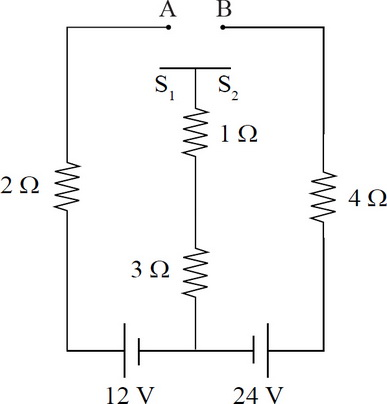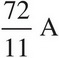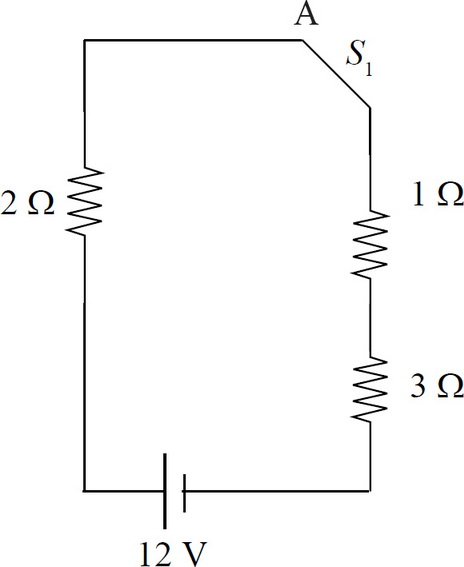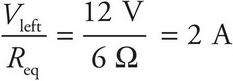# AP Physics 1 Question 297: Answer and Explanation

### Test Information

Question: 297

5. Question below refers to the below circuit diagram.If switch S1 is connected to point A but switch S2 is left unconnected, what is the current through the 1-Ω resistor?

• A. 0 A
• B. 2 A
• C. 3 A
• D.With S1 closed but S2 open, only the left-hand loop is connected. The resistors are in series, so the equivalent resistance is Req = 2 Ω + 1 Ω + 3 Ω = 6 Ω. The current through the circuits including the 1 Ω resistor is Ileft =.